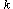Volume 26 • Number 2 • 2003
 • On a Class of Residually Finite Groups Bijan Taeri Abstract. Letbe positive integers andbe non-zero integers. We denote bythe class of groupsin which, for every subsetofof cardinality, there exist a subset, with,, and a function, withsuch thatwhere,. The classis defined exactly as, with additional conditions "whenever, where". Let G be a finitely generated residually finite group. Here we prove that (1) If, thenhas a normal nilpotent subgroupwith finite index such that the nilpotency class ofis bounded by a function of, where, is the torsion subgroup of. (2) Ifbegenerated, thenhas a normal nilpotent subgroupwhose index and the nilpotency class are bounded by a function of. Full text: PDF